# Combinations 6

6 purses
9 flaps
12 straps
Every combination must include 1 purse, 1 flap, and 1 strap. How many are possible combinations?

Result

n =  108

#### Solution:

$n=\dfrac{ 6 \cdot \ 9 \cdot \ 12 }{ 3! }=108$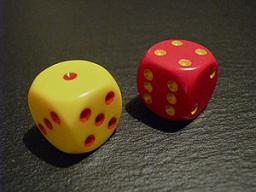Our examples were largely sent or created by pupils and students themselves. Therefore, we would be pleased if you could send us any errors you found, spelling mistakes, or rephasing the example. Thank you!

Leave us a comment of this math problem and its solution (i.e. if it is still somewhat unclear...):Math studentMatematik
Why is it not right answer 6*9*12?

4 months ago  1 LikeMath student
it is 108 because 6*9*12÷3!Tips to related online calculators
Would you like to compute count of combinations?

#### You need to know the following knowledge to solve this word math problem:

We encourage you to watch this tutorial video on this math problem:

## Next similar math problems:

1. Possible combinations - word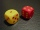How many ways can the letters F, A, I, R be arranged?How many ways can we thread 4 red, 5 blue, and 6 yellow beads onto a thread?
3. Three workplacesHow many ways can we divide nine workers into three workplaces if they need four workers in the first workplace, 3 in the second workplace and 2 in the third?
4. VCP equationSolve the following equation with variations, combinations and permutations: 4 V(2,x)-3 C(2,x+ 1) - x P(2) = 0
5. CombinationsIf the number of elements increase by 3, it increases the number of combinations of the second class of these elements 5 times. How many are the elements?
6. LunchSeven classmates go every day for lunch. If they always come to the front in a different order, will be enough school year to take of all the possibilities?
7. Five letters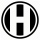How many ways can five letters be arranged?
8. Bookshelf and books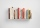How many ways can we place 7 books in a bookshelf?
9. ClassroomOf the 26 pupils in the classroom, 12 boys and 14 girls, four representatives are picked to the odds of being: a) all the girls b) three girls and one boy c) there will be at least two boys
10. Practice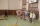How many ways can you place 20 pupils in a row when starting on practice?
11. Boys and girlsThere are eight boys and nine girls in the class. There were six children on the trip from this class. What is the probability that left a) only boys b) just two boys
12. Cancel fractionsCompress the expression of factorial: (n+6)!/(n+4)!-n!/(n-2)!
13. Desks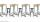A class has 20 students. The classroom consists of 20 desks, with 4 desks in each of 5 different rows. Amy, Bob, Chloe, and David are all friends, and would like to sit in the same row. How many possible seating arrangements are there such that Amy, Bob,
14. Math logicThere are 20 children in the group, each two children have a different name. Alena and John are among them. How many ways can we choose 8 children to be among the selected A) was John B) was John and Alena C) at least one was Alena, John D) maximum one
15. Elements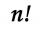If the number of elements is decreased by two the number of permutations is decreased 30 times. How many elements are?
16. PrizeHow many ways can be rewarded 9 participants with the first, second and third prize in a sports competition?
17. Value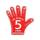Find the value of the expression: 6!·10^-3